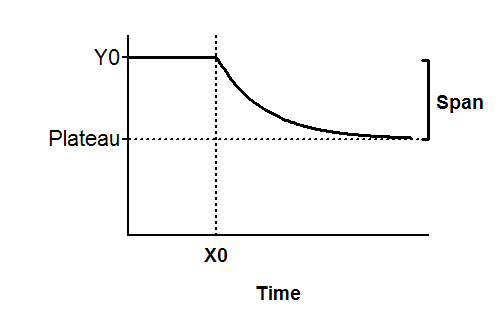## Please enable JavaScript to view this site.

 Equation: Plateau followed by one phase decay

## Introduction

In the standard one-phase decay equation, the decay starts at time 0. This equation is used when you measure a baseline for a while, then do some experimental intervention that starts the decay at some time X0.

## Entering data

Create an XY data table. Enter time into X, and response (binding, concentration ..) into Y. If you have several experimental conditions, place the first into column A, the second into column B, etc.

After entering data, click Analyze, choose nonlinear regression, choose the panel of exponential equations, and choose Plateau followed by one phase decay.

## Consider constraining X0 and Plateau to a constant values

If you know the time at which you initiated the decay, you should constrain X0 to that value.

If you have subtracted off any background signal, then you know the curve has to plateau at Y=0. In this case, you should constrain the parameter Plateau to be a constant value equal to zero.

To constrain parameters to constant values, go to the Constrain tab of the nonlinear regression dialog, set the drop down next to the parameter name to "Constant equal to" and enter the value.

## Model

Y= IF( X<X0, Y0, Plateau+(Y0-Plateau)*exp(-K*(X-X0)))X0 is the time at which the decay begins. Often you will set that to a constant value based on your experimental design, but otherwise Prism can fit it. It is expressed in the same time units as X.

Y0 is the average Y value up to time X0. It is expressed in the same units as Y,

Plateau is the Y value at infinite times, expressed in the same units as Y.

K is the rate constant, expressed in reciprocal of the X axis time units. If X is in minutes, then K is expressed in inverse minutes.

Tau is the time constant, expressed in the same units as the X axis. It is computed as the reciprocal of K.

Half-life is in the time units of the X axis. It is computed as ln(2)/K.

Span is the difference between Y0 and Plateau, expressed in the same units as your Y values.